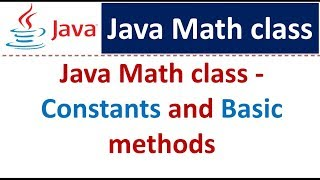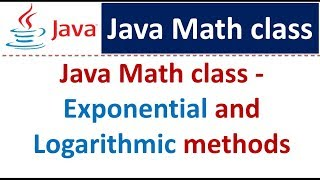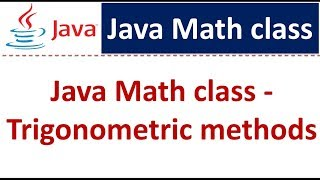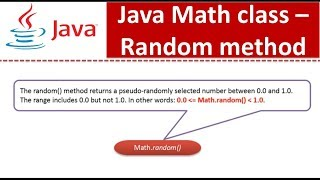java.math package tutorials

•WatchJava Math class (Constants and Basic methods) | Java Tutorial
•WatchJava Tutorial : Java Math class (Exponential and Logarithmic methods)
•WatchJava Math class (Trigonometric methods) | Java Tutorial
•WatchJava Math class (Random method) | Java Tutorial